GFG App
Open AppBrowser
Continue

# 8085 program to swap two 8-bit numbers

Problem: Write an assembly language program to swap two 8-bit numbers stored in an 8085 microprocessor.

Assumption: Suppose there are two 8-bit numbers. One 8-bit number is stored at location 2500 memory address and another is stored at location 2501 memory address. Let 05 be stored at location 2500 and 06 be stored at location 2501 (not necessarily, can be any two 8-bit numbers).

Example –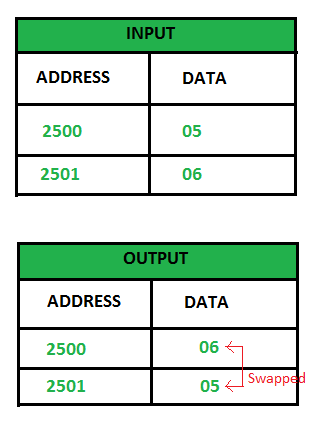Algorithm –

1. Load accumulator with the content of anyone location (either 2500 or 2501 or any given location).
2. Move the contents of the accumulator to any register (say B) so that another location’s content can be loaded to the accumulator and the previous data of the accumulator gets saved in the register.
3. Store the content of the accumulator in another location (data of 2501 to 2500).
4. Load accumulator with the content of register and then store it to another address location.

Program –

Explanation –

1. LDA 2500 – Load accumulator with the content of location 2500
2. MOV B, A – Copy content of accumulator to register B
3. LDA 2501 – Load accumulator with the content of location 2501
4. STA 2500 – Store content of accumulator to location 2500
5. MOV A, B – Copy content of register B to accumulator
6. STA 2501 – Store content of accumulator to location 2501
7. HLT – Terminates the program

Approach: 2  Using XRA Instruction :

We can use the XOR operation to swap 2 numbers

without using extra variables we know that we can swap x and y by using :

x = x ^ y

y = x ^ y

x = x ^ y

In the same way we can use registers to find solutions for the same. Implementation is provided below :

• Code + Output :

Initial Code + Location Counter as : 2050H and 2051H  . [2050H] = 4C and [2051H] =3E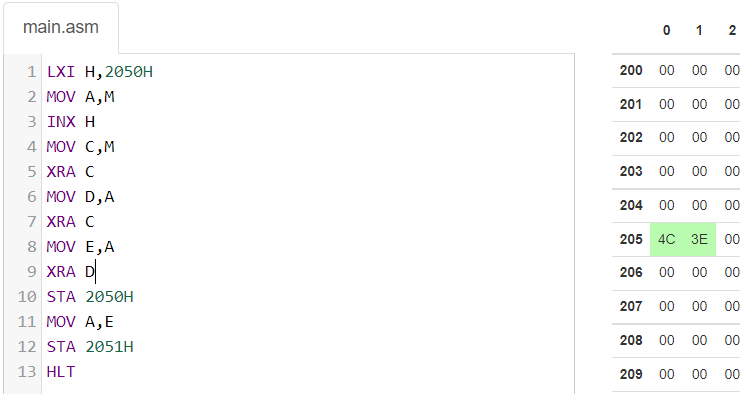Before Execution Data

Code after Assembling and Execution:

Swapping is done and location counters sets as [2050H] =3E and [2051H] = 4C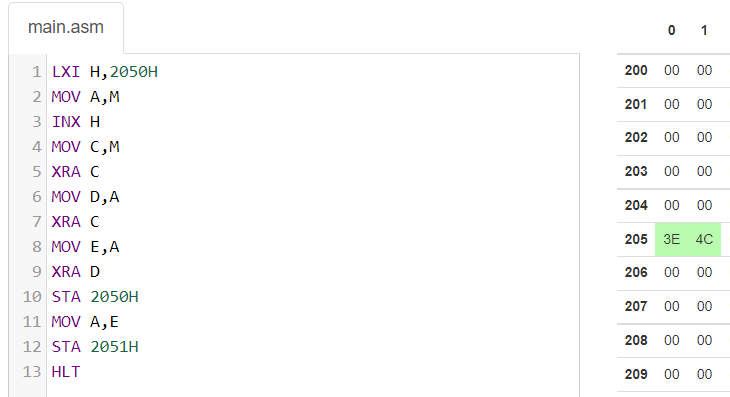After execution Swapped Data

In this way, with the use of XOR instruction available in the 8085 microprocessor, we can swap two 8-Bit numbers.

Approach : 3>> Using XCHG instruction :

• Before Exchange (Input Data Phase) : [2500H] = 1F , [2501H] = 9E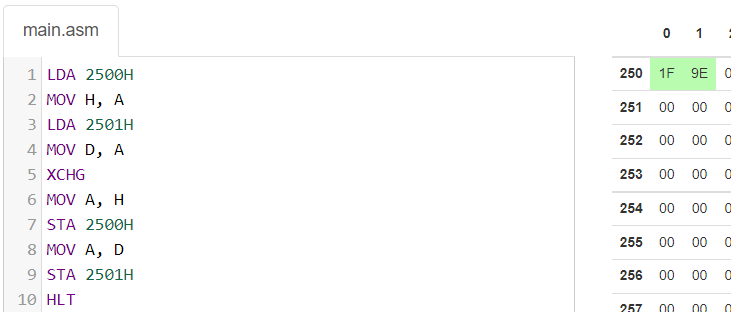• After Assembled and loaded program : Exchanged Data : [2500H] = 9E , [2501H] = 1F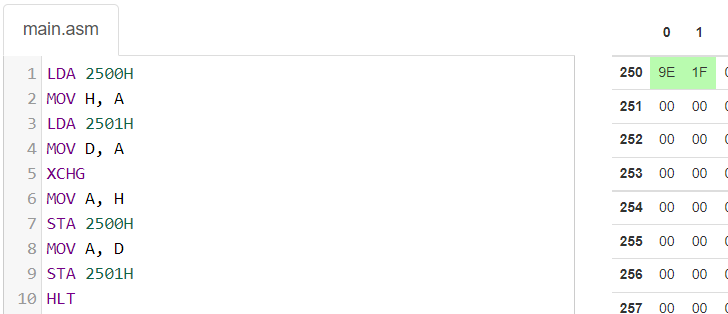Hence, In this way, we can swap any two 8-bit data using the above 3 approaches and by using different instructions.

My Personal Notes arrow_drop_up